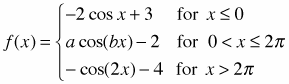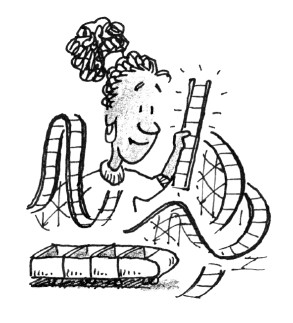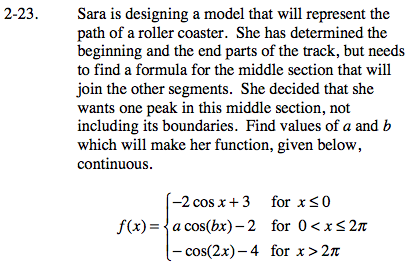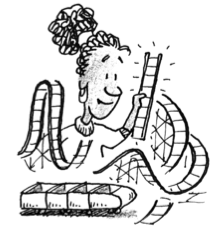### Home > CALC > Chapter Ch2 > Lesson 2.1.2 > Problem2-23

2-23.
1. Sara is designing a model that will represent the path of a roller coaster. She has determined the beginning and the end parts of the track, but needs to find a formula for the middle section that will join the other segments. She decided that she wants one peak in this middle section, not including its boundaries. Find values of a and b which will make her function, given below, continuous. To help you visualize this, use the 2-23 eTool (Desmos). Homework Help ✎2.Sara's roller coaster needs to be continuous. That means that the y-values at the boundary points (x = 0 and x = 2π) need to agree from the left and the right.

Determine the y-value of the roller coaster at x = 0: −2cos(0) + 3 = 1

Next, determine the y-value of the roller coaster at x = 2π: −cos(2(2π)) −4 = −5

We are ready to find the equation of the middle piece of the piecewise function. This piece has two unknowns, a and b. Fortunately, in Step 2 and Step 3, we found two values that exist on the middle equation, if the piecewise is continuous.
Write and solve a system for a and b:
1 = acos(bx) −2 when x = 0
−5 = acos(bx) −2 when x = 2π

Use the eTool below to dynamically adjust the graph.
Click the link at right for the full version of the eTool: Calc 2-23 HW eTool